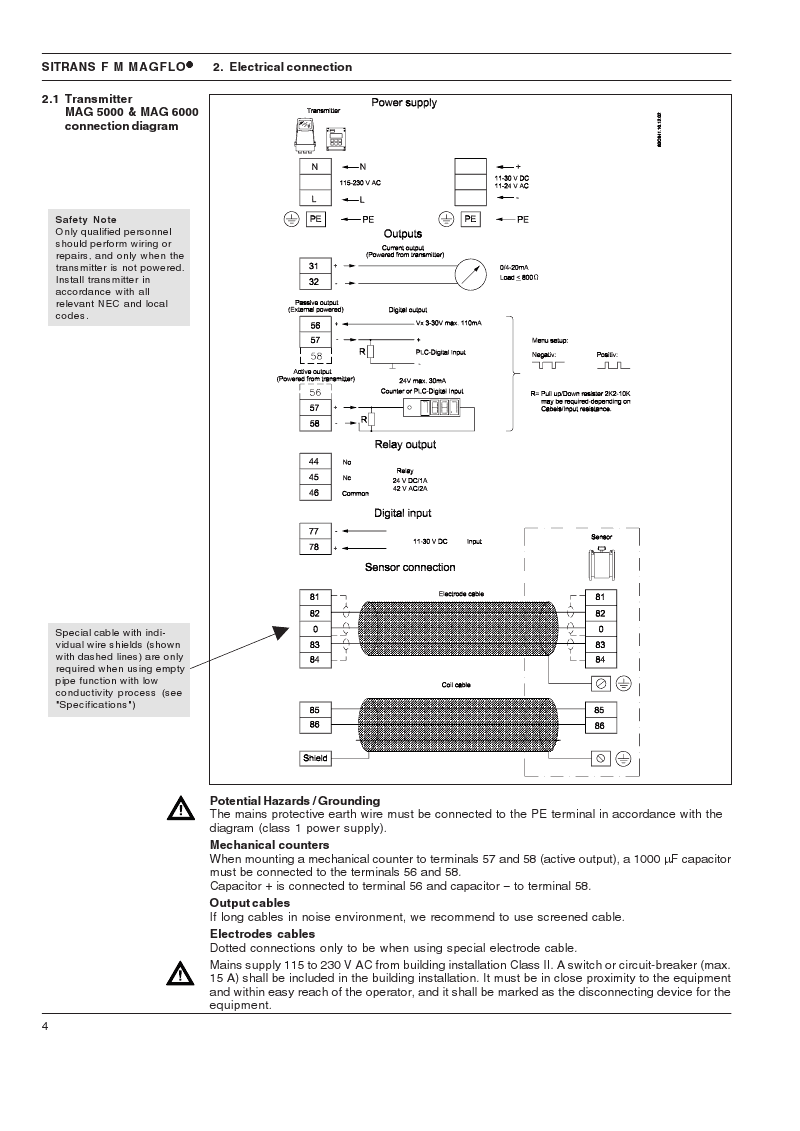# Mass flow meter

Hi! Mass flow meter's output variates from 4-20 mA. How i can see this variation on analog channel?

Regards Zee

Google knows me, now I can die in Peace (not soon I hope), knowing that I will live on forever in the form of old Arduino forum postings. Hum, that is a rather morbid thought. :~

First you need to determine if your mass flow meter is a 2 wire loop powered device, or does it provide the voltage source for the current loop from an internal DC power supply?

If it's loop powered most devices require at least a 12vdc source loop voltage and can utilize up to 48vdc source voltage, with 24vdc being the most common voltage used in loop powered 4-20 ma current loop devices. So assuming you are going to use a 12vdc source, wire the negative terminal of the 12vdc power supply to the arduino ground, wire a 250 ohm resistor from ground to a arduino analog input. Then run another wire from the same analog input pin to the negative terminal of the flow meter. Then finally run a wire from the flow meters positive terminal to the +12vdc power supply terminal. So when the flow meter is reading a 'zero' flow rate it will regulate the current to 4 ma, which when flowing through the 250 ohm resistor will cause a voltage drop of 1vdc with the arduino will read as about 205 'counts' . When the flow meter is running at 100% rated flow it will set the loop to 20 ma which will drop 5vdc across the resistor which the arduion will read as 1023 counts. You can use the arduino map() command to scale the 205-1023 flow measurement range to whatever flow units range the meter is set up for.

Just be sure to never let either end of the 250 ohm resistor become disconnected from the arduino ground or the analog input pin, as that would result in the full loop voltage (+12vdc or higher) to be seen at the arduino analog input pin which will damage the pin and perhaps the whole chip

That help?

Lefty

Actually i m working on CNG dispenser... The problem i m facing is that i cant determine any change in mass flow meter, as the CNG is filling in car.

Thanx lefty i got u tomorrow i will implement this on CNG dispenser n then let u know... Thanx again

Regards, Zee

Hello Brother…
if it is really changing current from 4-20mA and you only need to plot this change using Arduino then i think it must be simple, give that data pin to an analog pin 0 and put this program into your Arduino and open up the serial monitor so it will plot you the change of 4 to 20 into 0 to 1024 pieces as ADC in Arduino is 10 bit.

``````/*
Circuit:
> Data pin connected to analog pin 0.
> Ground on CNG Board is connected to Ground of Arduino
*/

int dataPin    = A0;
int dataValue = 0 ;
void setup()
{
// initialize serial communications at 9600 bps:
Serial.begin(9600);
}

void loop()
{
// print the results to the serial monitor:
Serial.print("Data = " );
Serial.print(dataValue);

// wait 10 milliseconds before the next loop
// for the analog-to-digital converter to settle
delay(10);
}
``````

Are there any 4-20mA devices that are NOT loop powered? I though that was the whole point of 4-20mA. The 4mA floor is the minimum power required for the device to output "zero".

Yes there are. In the process control world they are sometimes called "4-wire transmitters", where two of the wires are for a input power supply for the transmitter, and the other two wires are the now 'powered' 4-20ma current loop output. This allows measurement of the loop at the 'receiver' end to just use a passive 250 ohm resistor, with one of the wires also grounded to the measurement systems ground.

So a 2-wire 4-20ma transmitter device requires that the loop is powered externally, while a 4-wire transmitter can power the loop.

Lefty

retrolefty: Yes there are. In the process control world they are sometimes called "4-wire transmitters", where two of the wires are for a input power supply for the transmitter, and the other two wires are the now 'powered' 4-20ma current loop output. This allows measurement of the loop at the 'receiver' end to just use a passive 250 ohm resistor, with one of the wires also grounded to the measurement systems ground.

So a 2-wire 4-20ma transmitter device requires that the loop is powered externally, while a 4-wire transmitter can power the loop.

Lefty

So in 4 wire system for arduino is the positive is connect to 5v pin and negative wire to 250ohm and then to analog pin? thanks

So in 4 wire system for arduino is the positive is connect to 5v pin and negative wire to 250ohm and then to analog pin? thanks

That doesn't sound correct at all. Without a link to a specific 4-wire device you are talking about it's hard to speculate.

Lefty

retrolefty: That doesn't sound correct at all. Without a link to a specific 4-wire device you are talking about it's hard to speculate.

LeftyI am using the first one pin 31 32 current output powered from transmitter. Please Help, Thank You.